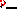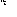# MINLPLib

### A Library of Mixed-Integer and Continuous Nonlinear Programming Instances

#### Instance: spring

 Formatsⓘ ams gms mod nl osil Primal Bounds (infeas ≤ 1e-08)ⓘ 0.84624567 p1 ( gdx sol ) (infeas: 3e-15) Other points (infeas > 1e-08)ⓘ Dual Boundsⓘ 0.84624567 (ANTIGONE)0.84624567 (BARON)0.84624567 (COUENNE)0.84624567 (LINDO)0.84624567 (SCIP) Referencesⓘ Sandgren, E, Nonlinear Integer and Discrete Programming in Mechanical Design Optimization, Journal of Mechanical Design, 112:2, 1990, 223-229. Sourceⓘ modified MacMINLP model spring.mod, modified GAMS Model Library model spring Applicationⓘ Coil Compression String Design Added to libraryⓘ 01 May 2001 Problem typeⓘ MINLP #Variablesⓘ 17 #Binary Variablesⓘ 11 #Integer Variablesⓘ 1 #Nonlinear Variablesⓘ 5 #Nonlinear Binary Variablesⓘ 0 #Nonlinear Integer Variablesⓘ 1 Objective Senseⓘ min Objective typeⓘ polynomial Objective curvatureⓘ indefinite #Nonzeros in Objectiveⓘ 3 #Nonlinear Nonzeros in Objectiveⓘ 3 #Constraintsⓘ 8 #Linear Constraintsⓘ 3 #Quadratic Constraintsⓘ 1 #Polynomial Constraintsⓘ 0 #Signomial Constraintsⓘ 3 #General Nonlinear Constraintsⓘ 1 Operands in Gen. Nonlin. Functionsⓘ div Constraints curvatureⓘ indefinite #Nonzeros in Jacobianⓘ 40 #Nonlinear Nonzeros in Jacobianⓘ 11 #Nonzeros in (Upper-Left) Hessian of Lagrangianⓘ 16 #Nonzeros in Diagonal of Hessian of Lagrangianⓘ 2 #Blocks in Hessian of Lagrangianⓘ 1 Minimal blocksize in Hessian of Lagrangianⓘ 5 Maximal blocksize in Hessian of Lagrangianⓘ 5 Average blocksize in Hessian of Lagrangianⓘ 5.0 #Semicontinuitiesⓘ 0 #Nonlinear Semicontinuitiesⓘ 0 #SOS type 1ⓘ 0 #SOS type 2ⓘ 0 Infeasibility of initial pointⓘ 9.059 Sparsity JacobianⓘSparsity Hessian of Lagrangianⓘ```\$offlisting
*
*  Equation counts
*      Total        E        G        L        N        X        C        B
*          9        6        0        3        0        0        0        0
*
*  Variable counts
*                   x        b        i      s1s      s2s       sc       si
*      Total     cont   binary  integer     sos1     sos2    scont     sint
*         18        6       11        1        0        0        0        0
*  FX      0
*
*  Nonzero counts
*      Total    const       NL      DLL
*         44       30       14        0
*
*  Solve m using MINLP minimizing objvar;

Variables  x1,x2,x3,i4,x5,x6,b7,b8,b9,b10,b11,b12,b13,b14,b15,b16,b17,objvar;

Binary Variables  b7,b8,b9,b10,b11,b12,b13,b14,b15,b16,b17;

Integer Variables  i4;

Equations  e1,e2,e3,e4,e5,e6,e7,e8,e9;

e1.. -(1.570796327 + 0.7853981635*i4)*x1*sqr(x2) + objvar =E= 0;

e2.. -x1/x2 + x5 =E= 0;

e3.. -((-1 + 4*x5)/(-4 + 4*x5) + 0.615/x5) + x6 =E= 0;

e4.. 2546.47908913782*x6*x5/sqr(x2) =L= 189000;

e5.. -6.95652173913044e-7*x5**3*i4/x2 + x3 =E= 0;

e6.. (2.1 + 1.05*i4)*x2 + 1000*x3 =L= 14;

e7..    x1 + x2 =L= 3;

e8..    x2 - 0.207*b7 - 0.225*b8 - 0.244*b9 - 0.263*b10 - 0.283*b11 - 0.307*b12
- 0.331*b13 - 0.362*b14 - 0.394*b15 - 0.4375*b16 - 0.5*b17 =E= 0;

e9..    b7 + b8 + b9 + b10 + b11 + b12 + b13 + b14 + b15 + b16 + b17 =E= 1;

* set non-default bounds
x1.lo = 0.414;
x2.lo = 0.207;
x3.lo = 0.00178571428571429; x3.up = 0.02;
i4.lo = 1; i4.up = 100;
x5.lo = 1.1;

Model m / all /;

m.limrow=0; m.limcol=0;
m.tolproj=0.0;

\$if NOT '%gams.u1%' == '' \$include '%gams.u1%'

\$if not set MINLP \$set MINLP MINLP
Solve m using %MINLP% minimizing objvar;

```

Last updated: 2019-11-29 Git hash: 4a97e4ed